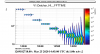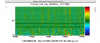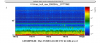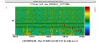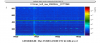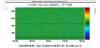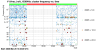Reports 1-1 of 1
Detector Characterisation (General)
verkindt - 0:38 Thursday 26 March 2020 (48810) Print this report
Detchar Hardware Injections

Using the frame file produced by N. Arnaud and copied here: /data/procdata/calibration/HInjection/input0/HI_20200325_v1.gwf
the following detchar injections have been done:
14:00 UTC : one set of injections, but with all amplitudes divided by 10
14:12 UTC: one set of injections, with the initial amplitudes
14:30 UTC: one set of injections (but not finished), with all amplitudes multiplied by 10
then, we tried to use the ITF out of earthquake mode, but ITF unlocked and it took some time to get it back to LN3.
16:40 UTC: one set of injections, with the initial amplitudes
16:50 UTC: one set of injections, with the initial amplitudes

A set of injections has a duration of 495 sec and is made of the following sine-gaussian glitches sampled at 10 kHz, with a width of 0.6 sec and a frequency f :
f=811 Hz : SNR=20,50,100,500 (4 injections, one every 15 sec)
f=409 Hz : SNR=20,50,100,500
f=211 Hz : SNR=20,50,100,500
f=129 Hz : SNR=20,50,100,500
f=73 Hz : SNR=20,50,100,500
f=47 Hz : SNR=20,50,100,500
f=31 Hz : SNR=20,50,100,500
f=19 Hz : SNR=20,50,100,500

Plot 1 shows the spectrogram of the input set of injections as read from the input frame file
Plot 2 shows the spectrogram of Hrec_hoft_raw_20000Hz (normalized by median value to better see the injected glitches) where can be seen the injections done with all amplitudes divide by 10
Plots 3 shows the spectrogram of Hrec_hoft_raw_20000Hz where can be seen the injections done at 14:12 UTC, with the initial amplitudes (plot 4 shows the same but normalized by median value).
Plot 5 shows the spectrogram of Hrec_hoft_raw_20000Hz where can be seen the injections done at 14:30 UTC with all amplitudes multiplied by 10. It confirms that too high SNR injections at high frequency induces a saturation (visible as a big glitch at low frequency).

Plots 6 and 7 shows the spectrograms (normalized by median) for the 16:40 UTC and 16:50 UTC injection sets. Plot 7 shows that the 16:50 injections were in noisier conditions.

Plot 8 shows the Omicron triggers seen over the time period of those detchar injections.

Images attached to this report